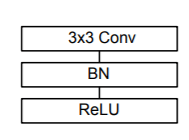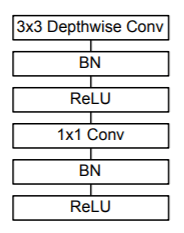# MobileNet Architecture

FREE BOOK -> Problems for the day before your Coding Interview (on Amazon)MobileNet model has 27 Convolutions layers which includes 13 depthwise Convolution, 1 Average Pool layer, 1 Fully Connected layer and 1 Softmax Layer.

In terms of Convolution layers, there are:

• 13 3x3 Depthwise Convolution
• 1 3x3 Convolution
• 13 1x1 Convolution

95% of the time is spent in 1x1 Convolution in MobileNet. This model was developed by Andrew G. Howard and other researchers from Google.

# Parameters of MobileNet

The standard MobileNet model has 4.2 Million parameters while smaller versions of MobileNet has 1.32 Million parameters. This is low compared to other standard Machine Learning models like:

Model Number of Parameters
AlexNet 60 Million
SqueezeNet 1.25 Million
VGG16 138 Million 😱

# Layers in order

The layers in order (from first to last) are as follows:

• 3x3 Convolution
• 3x3 Depthwise Convolution
• 1x1 Convolution
• 3x3 Depthwise Convolution
• 1x1 Convolution
• 3x3 Depthwise Convolution
• 1x1 Convolution
• 3x3 Depthwise Convolution
• 1x1 Convolution
• 3x3 Depthwise Convolution
• 1x1 Convolution
• 3x3 Depthwise Convolution
• 1x1 Convolution
• 3x3 Depthwise Convolution
• 1x1 Convolution
• 3x3 Depthwise Convolution
• 1x1 Convolution
• 3x3 Depthwise Convolution
• 1x1 Convolution
• 3x3 Depthwise Convolution
• 1x1 Convolution
• 3x3 Depthwise Convolution
• 1x1 Convolution
• 3x3 Depthwise Convolution
• 1x1 Convolution
• 3x3 Depthwise Convolution
• 1x1 Convolution
• Average Pool
• Fully Connected layer
• Softmax

# Distribution of Parameters

Model Number of Parameters
1x1 Convolution 74.59%
Fully Connected 24.33%
3x3 Depthwise Convolution 1.06%
3x3 Convolution 0.015%
Average Pool 0.005%

Hence, nearly 75% of the parameters are a part of Convolution.

# Detailed architecture of MobileNet

If you notice carefully, there are two basic units:

• 3x3 Convolution
• 3x3 Depthwise Convolution followed by 1x1 Convolution

Unit 1:

3x3 Convolution is followed by Batch Normalization and ReLU activation. This is the first layer of MobileNet and has a kernel dimension of 3x3x3x32. It takes input of dimension 224x224x3 and the output is of dimension 112x112x32.This unit 1 is present at the beginning only and hence, is used only once.

Unit 2:

3x3 depthwise convolution is followed by batch normalization and ReLU activation. This sub-unit is followed by 1x1 Convolution which is, similarly, followed by Batch Normalization and ReLU activation. Hence, the sequence of these two sub-units creates our second unit. Let us denote this as Unit2.1 and Unit2.2 respectively.This unit is present multiple times and the input and filter size (except kernel size) vary along with the filter values.

Layer Filter Dimension Stride Input size
Unit1 (3x3) 3 x 3 x 3 x 32 2 224 x 224 x 3
Unit2.1 3 x 3 x 32 1 112 x 112 x 32
Unit2.2 1 x 1 x 32 x 64 1 112 x 112 x 32
Unit2.1 3 x 3 x 64 2 112 x 112 x 64
Unit2.2 1 x 1 x 64 128 1 56 x 56 x 64
Unit2.1 3 x 3 x 128 1 56 x 56 x 128
Unit2.2 1 x 1 x 128 x 128 1 56 x 56 x 128
Unit2.1 3 x 3 x 128 2 56 x 56 x 128
Unit2.2 1 x 1 x 128 x 256 1 28 x 28 x 128
Unit2.1 3 x 3 x 256 1 28 x 28 x 256
Unit2.2 1 x 1 x 256 x 256 1 28 x 28 x 256
Unit2.1 3 x 3 x 256 2 28 x 28 x 256
Unit2.2 1 x 1 x 256 x 512 1 14 x 14 x 256
Unit2.1 3 x 3 x 512 1 14 x 14 x 512
Unit2.2 1 x 1 x 512 x 512 1 14 x 14 x 512
Unit2.1 3 x 3 x 512 1 14 x 14 x 512
Unit2.2 1 x 1 x 512 x 512 1 14 x 14 x 512
Unit2.1 3 x 3 x 512 1 14 x 14 x 512
Unit2.2 1 x 1 x 512 x 512 1 14 x 14 x 512
Unit2.1 3 x 3 x 512 1 14 x 14 x 512
Unit2.2 1 x 1 x 512 x 512 1 14 x 14 x 512
Unit2.1 3 x 3 x 512 1 14 x 14 x 512
Unit2.2 1 x 1 x 512 x 512 1 14 x 14 x 512
Unit2.1 3 x 3 x 512 2 14 x 14 x 512
Unit2.2 1 x 1 x 512 x 1024 1 7 x 7 x 512
Unit2.1 3 x 3 x 1024 2 7 x 7 x 1024
Unit2.2 1 x 1 x 1024 x 1024 1 7 x 7 x 1024
AvgPool 7 x 7 1 7 x 7 x 1024
FC 1024 x 1000 1 1 x 1 x 1024
Softmax - 1 1 x 1 x 1000